TitleCollege Algebra
Tutorial 48: Practice Test on Tutorials 42 - 47Learning Objectives

 After completing this tutorial, you should be able to: Take a test on topics covered in tutorials 42 - 47 in this website.

 Special Notes about Tutorial 48: I can not guarantee you will pass your test after going though any of the tutorials in this website or this practice test. However, it will definitely help you to better understand the topics covered better. Disclaimer: WTAMU and Kim Seward are not responsible for how a student does on any test or any class for any reason including not being able to access the website due to any technology problems.  There are no videos on this page.Introduction

It is important to note that chances are I'm not your math instructor. You need to check with your math teacher as to things like when your next math test is and what it covers.  It may cover more material on the test than what is in this practice test.  Just note that there are other practice tests at this website. So, after finding out what is on your test (if you have one) do the practice test(s) problems that go with the test you are preparing for.  If you are not in a class or are not having a test soon, this practice test is still good practice to go through and check to make sure you are understanding this material before moving on - kind of like a spot check.  The material on this practice test goes with Tutorial 42: Exponential Functions, Tutorial 43: Logarithmic Functions,  Tutorial 44: Logarithmic Properties, Tutorial 45: Exponential Equations, and Tutorial 46: Logarithmic Equations.

Note that I will be adding on Tutorial 47 later.

Also note that your teacher may word the problems on their test a little differently, may have some different kinds of problems, or may have a different number of problems than what is in this practice test.  Again, since I'm probably not your math instructor, I don't know exactly how your teacher will set up your math test.  Just note that these problems will help you build an understanding of the concepts presented and the terms used in math problems.  If you have an understanding of the problems instead of just memorizing them, then you should do fine on these concepts, no matter how the test is set up.

 Steps to Studying for a Math Test
1. Work through problems.  If you are in a class, you should have done this on completion of any homework you have done.  For anyone,  you can accomplish this by doing the practice problems found in each tutorial.

2. Check work on problems.  The practice problems in each tutorial have links to the answers to them so you can instantly check how you are doing.  Also, in most math books, the odd answers are found in the back of the book.

3. Review concepts.  Whether you got the problems right or wrong, make sure you review over them.  If you did get a problem wrong, make sure you either review that concept in it's respective tutorial or ask your math teacher about it.  If you don't ask about a problem before a test,  you are going to kick yourself when it comes up on the test.

4. Work through problems as if you were taking the test - no notes, book, webpages, etc.  This practice test is a perfect way to do that. After taking this practice test, check your answers by clicking on the link to the answer key found at the bottom of the practice test (before the 'need extra help on these topics' section)

 During the Test

It is to your benefit to show as much of the work as possible on the problems that have several steps involved.

Make sure that you read the directions carefully,  you wouldn't believe how many points get taken off  math tests for people not following directions.

Pace yourself.  You do not have to be the first one done to do well on the test.  Do not panic if there is still time left to take the test and others are turing it in.  Sometimes that means they do not know the material and left some of the answers blank.  Do not worry about anyone else but yourself.

Don't rush through a problem.  Another thing that math teachers take points off for are careless mistakes made by people that rush through a problem.  When those students get their tests back, they bonk themselves on the head at some of the things that got counted wrong,  things that they knew how to do.

Remember to breathe!!!!  I know some of you are scared to death at the thought of having to take a math test of any kind.  For you guys, try to relax and don't forget to breathe.  (Even if you aren't scared to take a math test,  it is probably a good idea to remember to breathe,  I wouldn't want you to pass out during the test). If it feels like your brain has left the building during your test, just close your eyes and breathe in and out and in and out and your brain will return.

Good luck on your test.  If you are taking a math test soon, don't panic, you are going to do great!!!Practice Test

 Problems 1a - 1b: Graph the following functions.

 1a.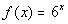1b.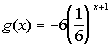Problem 2a:   Find the 1) compound amount AND 2) the compound interest for the given investment and rate.

 2a. \$25000 for 21 years at an annual rate of 3.25% compounded quarterly.

 Problem 3a: Find the accumulated value for the given investment and  rate.

 3a. \$4525 that is compounded continuously for 12 years at an interest rate of 9 ½ %.

 Problems 4a - 4b: Express the given logarithmic equation exponentially.

 4a.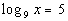4b.Problems 5a - 5b:  Express the given exponential equation in a logarithmic form.

 5a.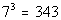5b.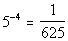Problems 6a - 6b:   Evaluate the given log function without using a calculator.

 6a.6b.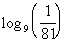Problems 7a - 7b: Graph the following functions.

 7a.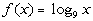7b.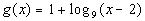Problems 8a - 8b:   Evaluate the given expression without the use of a calculator.

 8a.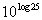8b.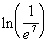Problems 9a - 9b:  Expand each logarithmic expression as much as possible.  Evaluate without a calculator where possible.

 9a.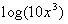9b.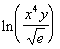Problems 10a - 10b:  Condense each logarithmic expression into one  logarithmic expression.  Evaluate without a calculator  where possible.

 10a.10b.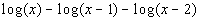Problems 11a - 11c:    Solve the given exponential equation.  Round your answer to two decimal places.

 11a.11b.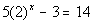11c.Problems 12a - 12b:   Solve the logarithmic equation.  Round your answer to two decimal places.

 12a.12b.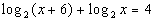Need Extra Help on these Topics?

The following are web pages that can assist you in the topics that were covered on this page:

Go to Get Help Outside the Classroom found in Tutorial 1: How to Succeed in a Math Class for some more suggestions.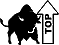Last revised on March 25, 2011 by Kim Seward.